# The shop

The shop has 3 hectoliters of water. How many liter bottles is it?

Result

n =  300

#### Solution:Leave us a comment of example and its solution (i.e. if it is still somewhat unclear...):Be the first to comment!#### To solve this verbal math problem are needed these knowledge from mathematics:

Do you know the volume and unit volume, and want to convert volume units?

## Next similar examples:

1. Infants water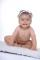From the tank, which has 10 hectoliters of water for infants, went 580 liters at mornening and 340 liters in the afternoon. How many liters left in the tank?
2. Cylindrical tank 2If a cylindrical tank with volume is used 12320cm raised to the power of 3 and base 28cm is used to store water. How many liters of water can it hold?
3. Water 31Richard takes 3 1/6 liters of water before noon and 2 3/5 liters of water after noon. How many litres of water does Richard consume a day ?
4. Tank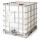How many minutes does it take to fill the tank to 25 cubic meters of water filled 150hl per hour?
5. AquariumAquarium is rectangular box with square base containing 76 liters of water. Length of base edge is 42 cm. To what height the water level goes?
6. AquariumAquarium is cube with edge 45 cm. How much water can fit in there?
7. Wood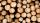Wood density is 0.6 g/cm3. How many kilograms weight 1 m3 of wood?
8. Caleb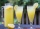Caleb is making lemonade for a party. He has 5 gallons of lemonade. He is putting 1/3 cubic inch of lemonade in a cup for each guest. How many guests are going to be at the party?
9. RecipeA recipe requires 2 pounds of flour. If a chef wants to triple the recipe, how many ounces of flour will be needed?
10. HoursThe lesson lasts 45 minutes. For the week, students have 18 lesson hours. How many are the actual hours?
11. Milk bill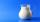Mrs Tara buys 2 liters of milk daily. If 1 liter of milk cost \$0.27. What will be her milk bill for 30 days.
12. AreaCalculate: ?
13. Jill's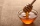Jill's mom stored some of the honey in a container that held 3/4 of a gallon. She used half of this amount to sweeten tea. How much honey, in cups was used in the tea. Express your answers in cups.
14. Fraction and a decimalWrite as a fraction and a decimal. One and two plus three and five hundredths
15. In fractionsAn ant climbs 2/5 of the pole on the first hour and climbs 1/4 of the pole on the next hour. What part of the pole does the ant climb in two hours?
16. LineCan we construct a line segment, if we know: center
17. SimplifySimplify the following problem and express as a decimal: 5.68-[5-(2.69+5.65-3.89) /0.5]